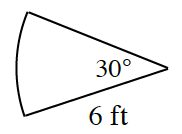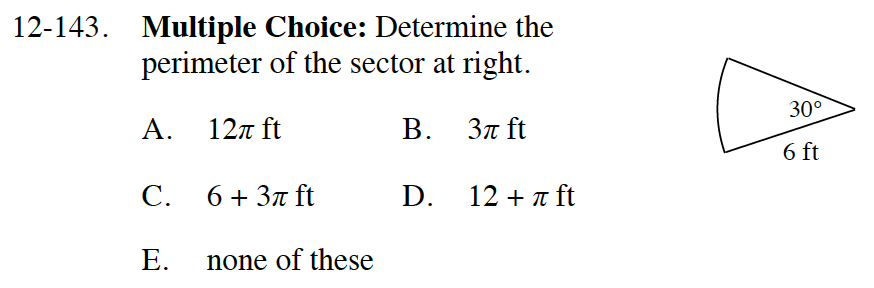### Home > INT2 > Chapter 12 > Lesson 12.2.4 > Problem12-143

12-143.
1. 12-143.Multiple Choice: Determine the perimeter of the sector at right. Homework Help ✎

2.  A. 12π ft B. 3π ft C. 6 + 3π ft D. 12 + π ft E. none of theseRemember that the perimeter of a sector is the sum of all the sides, including the arc length.

$\text{Recall the formal for the arc length: }\frac{m\angle }{360\degree}(2\pi r)$